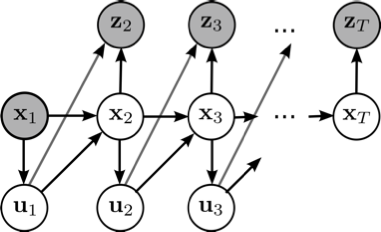# Probabilistic Machine Learning (RO5101 T)Univ.-Prof. Dr. Elmar Rueckert was teaching this course at the University of Luebeck in the winter semester in the years 2019 and 2020.

Teaching Assistant:
Nils Rottmann, M.Sc.

Language:
English only

## Course Details

Some remarks on the UzL Module idea: The lecture Probabilistic Machine Learning belongs to the Module Robot Learning (RO4100). In the winter semester, Prof. Dr. Elmar Rueckert is teaching the course Probabilistic Machine Learning (RO5101 T). In the summer semester, Prof. Dr. Elmar Rueckert is teaching the course Reinforcement Learning (RO5102 T). Important: Due to the study regulations, students have to attend both lectures to receive a final grade. Thus, there will be only a single written exam for both lectures. You can register for the written exam at the end of a semester.

## Important dates

• Written exam: 04. February 2021 (2nd appointment 04.03.2021)
• Assignment I: Freitag, 11. Dezember 2020, 23:00
• Assignment II: Freitag, 22. Januar 2021, 23:00

## The course topics are

1. Introduction to Probability Theory (Statistics refresher, Bayes Theorem, Common Probability distributions, Gaussian Calculus).
2. Linear Probabilistic Regression (Linear models, Maximum Likelihood, Bayes & Logistic Regression).
3. Nonlinear Probabilistic Regression (Radial basis function networks, Gaussian Processes, Recent research results in Robotic Movement Primitives, Hierarchical Bayesian & Mixture Models).
4. Probabilistic Inference for Filtering, Smoothing and Planning (Classic, Extended & Unscented Kalman Filters, Particle Filters, Gibbs Sampling, Recent research results in Neural Planning).
5. Probabilistic Optimization (Stochastic black-box Optimizer Covariance Matrix Analysis Evolutionary Strategies & Natural Evolutionary Strategies, Bayesian Optimization).

## The learning objectives / qualifications are

• Students get a comprehensive understanding of basic probability theory concepts and methods.
• Students learn to analyze the challenges in a task and to identify promising machine learning approaches.
• Students will understand the difference between deterministic and probabilistic algorithms and can define underlying assumptions and requirements.
• Students understand and can apply advanced regression, inference and optimization techniques to real world problems.
• Students know how to analyze the models’ results, improve the model parameters and can interpret the model predictions and their relevance.
• Students understand how the basic concepts are used in current state-of-the-art research in robot movement primitive learning and in neural planning.

## Interactive Online Lectures

In the lecture, Prof. Rueckert is using a self made lightboard to ensure an interactive and professional teaching environment. Have a look at the post on how to build such a lightboard. Here is an example recording.

## Requirements

Strong statistical and mathematical knowledge is required beforehand. It is highly recommended to attend the course Humanoid Robotics (RO5300) prior to attending this course. The students will also experiment with state-of-the-art machine learning methods and robotic simulation tools which require strong programming skills.

## Course dates & materials

22.10.2020VOAn Introduction to the Probabilistic Machine Learning (PML) lectureSlides
05.11.2020VO Random Variables, Fundamental Rules, Fundamental Distributions, Information TheoryIntersting Notes on Frequentist vs Bayesian by Jeremy Orloff and Jonathan Bloom, Visual Introduction to Probability Theory
12.11.2020VOInformation Theory, ML Overview
A gentle Introduction to Information Theory, Paper on using Similarity Measures to compare distributions, Lightboard Tutorial on deriving the Bayes Rule
19.11.2020VOLinear Regression
Human femur bone Regression Study , Slides
26.11.2020VOML Basics, Over- Underfitting, Cross validatin, Probabilistic Linear RegressionSummary of the lecture content
03.12.2020VOOverview linear regression, predictive distribution, parametric vs. non-parametric models, LWRSlides
10.12.2020VONon-linear Probabilistic Regression, Gaussian ProcessesSlides
07.01.2021VOMeta Learning for GPs, Markov Models, MMs Examples for PlanningSlides
21.01.2021VOProbabilistic Time Series ModelsMatlab Probabilistic Timer Series Model Demo, Slides to Probabilistic Time Series Models
28.01.2021VOQ & A for the final exam.Slides
04.02.2021 (CANCELLED)CANCELLED ExamCANCELLED: Old date of the written exam. CANCELLED
04.03.2021 (TENTATIVE)Planned als Online Moodle Exam, first appointmentDate of the written online exam. Details will be sent via Moodle to all students by Feb. 15th 2021